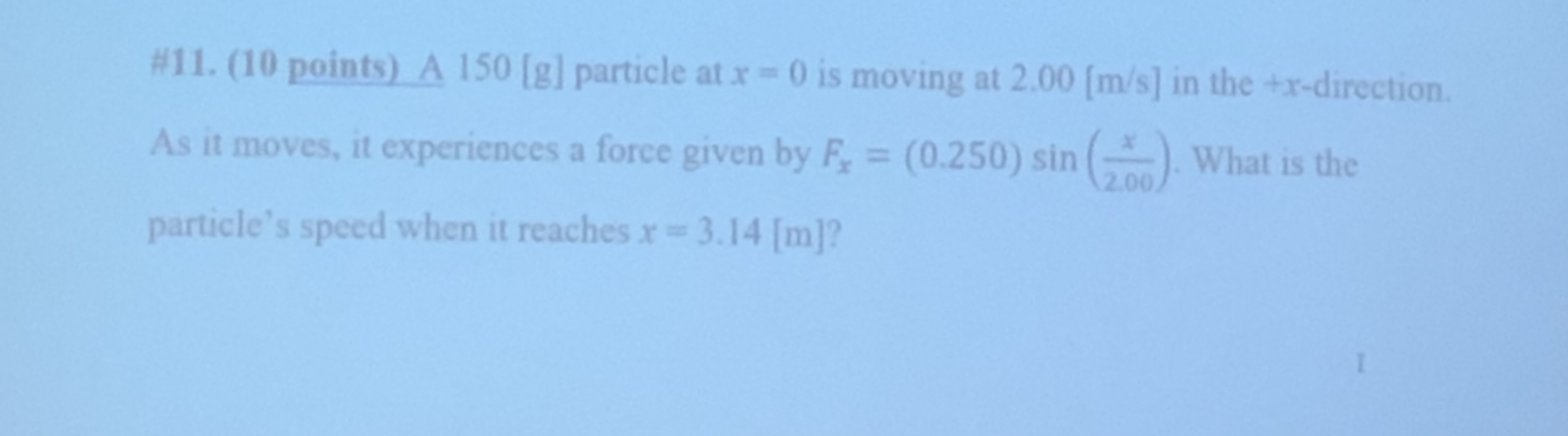# #11. (10 points) A 150 [g] particle at x 0 is moving at 2.00 [m/s] in the +x-directionAs it moves, it experiences a force given by F (0.250) sin ( What is the2.00particle's speed when it reaches r= 3.14 [m]?

Question
64 views

Need help on a review questionhelp_outlineImage Transcriptionclose#11. (10 points) A 150 [g] particle at x 0 is moving at 2.00 [m/s] in the +x-direction As it moves, it experiences a force given by F (0.250) sin ( What is the 2.00 particle's speed when it reaches r= 3.14 [m]? fullscreen
check_circle

Step 1

the work done is equal to the change in kinetic energy,

the work done is,

Step 2

integrate the above equation by substituting the given values,

Step 3

the change in kinetic ...

### Want to see the full answer?

See Solution

#### Want to see this answer and more?

Solutions are written by subject experts who are available 24/7. Questions are typically answered within 1 hour.*

See Solution
*Response times may vary by subject and question.
Tagged in

### Kinematics